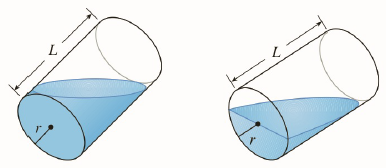Chapter 5.P, Problem 4P

Chapter
Section
Textbook Problem

# A cylindrical glass of radius r and height L is filled with water and then tilted until thewater remaining in the glass exactly covers its base.(a) Determine a way to “slice” the water into parallel rectangular cross-sections and then set up a definite integral for the volume of the water in the glass.(b) Determine a way to “slice” the water into parallel cross-sections that are trapezoids and then set up a definite integral for the volume of the water.(c) Find the volume of water in the glass by evaluating one of the integrals in part (a) or part (b).(d) Find the volume of the water in the glass from purely geometric considerations.(e) Suppose the glass is tilted until the water exactly covers half the base. In what direction can you “slice” the water into triangular cross-sections? Rectangular cross-sections? Cross-sections that are segments of circles? Find the volume of water in the glass.To determine

(a)

A way to “slice” the water into parallel rectangular cross-sections and thenset upa definite integral for the volume of the water in the glass

Explanation

Concept:

Let S be a solid that lies between y=a  and y=b. If the cross sectional area of S in the plane Py through y and perpendicular to the y axis, is A(y), where A  is a continuous function, then volume of S is

V=abAydy

Calculation:

Consider the diagram below.

In order to take rectangular cross sections, slice the water in xz  plane.

Viewing from FV forms the circle of radius r in xy plane, which can be considered as the width of rectangle, and viewing from SV forms the triangle, which can be considered as the length of the rectangle.

Equation of the circle passing through the origin:

x2+y2=r2

x2=r2-y2

x=r2-y2

Now width of rectangle is 2x.

w=2x=2r2-y2

To calculate length BC, consider PQR and PBC

To determine

(b)

A way to slice water into parallel cross-sections and then set up a definite integral for the volume of the water in the glass

To determine

(c)

To find:

Volume of water

To determine

(d)

To find:

Volume of water

To determine

(e)

To find:

Volume of water

### Still sussing out bartleby?

Check out a sample textbook solution.

See a sample solution

#### The Solution to Your Study Problems

Bartleby provides explanations to thousands of textbook problems written by our experts, many with advanced degrees!

Get Started

#### In Exercises 2340, find the indicated limit. 35. limx2x+2

Applied Calculus for the Managerial, Life, and Social Sciences: A Brief Approach

#### Evaluate: 123(52)

Elementary Technical Mathematics

#### Is it true that f(g+h)=fg+fh?

Single Variable Calculus: Early Transcendentals

#### Multiply and reduce to lowest terms. a. b.

Contemporary Mathematics for Business & Consumers

#### The radius of convergence of is: 1 3 ∞

Study Guide for Stewart's Single Variable Calculus: Early Transcendentals, 8th